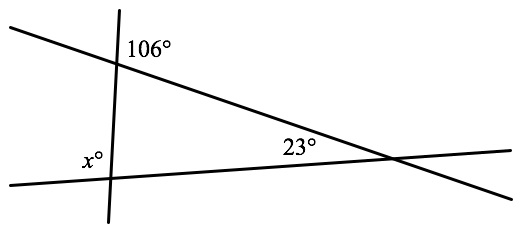Can you please do #20 from the no calculator section in test 5?

Sure. Here’s (basically) the image you’re given:To find the value of x, you need to remember that the angles that make up straight lines and the angles that make up triangles will both sum to 180°. You’ve got a straight line (marked in red below) that helps you calculate that the upper left angle in the triangle is 180° – 106° = 74°.From there, you can calculate the measure of the third angle in the triangle: 180° – 23° – 74° = 83°. Just one more 180° straight line to find the value of x:= 180º – 83°
= 97°

A note since I’m sure someone would bring it up in the comments if I didn’t: another way to go here is the Exterior Angle Theorem, which states that the measure of an exterior angle of a triangle (the 106° angle in this case) equals the sum of the measures of the nonadjacent interior angles (the 23° and the 83°). You can use that theorem as a “shortcut” to find the 83° angle in one fewer step if you like. 🙂